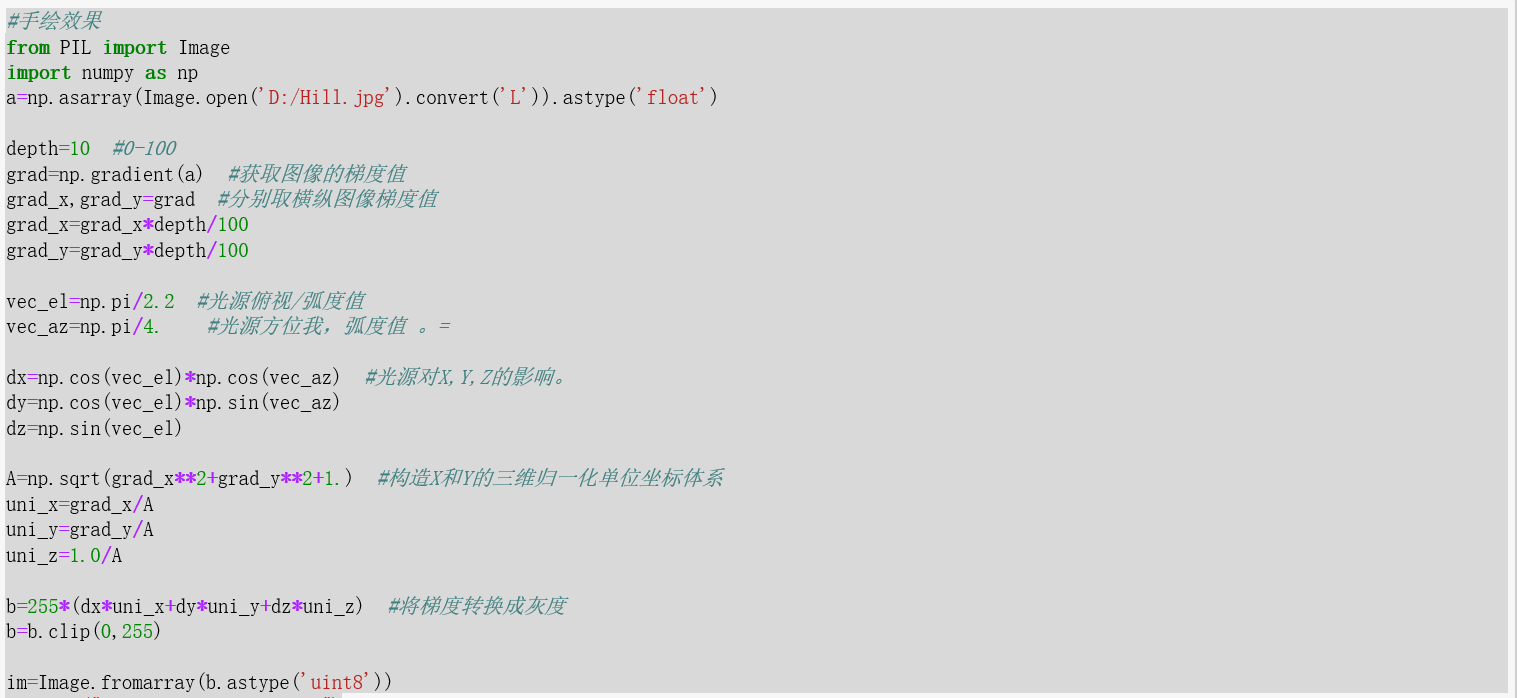2016-03-30 10:07:07 Trent1985 阅读数 2886
• ###### 手绘漫画人体结构与比例入门基础教程

课程详细介绍了插画常用的软件SAI、PS，数位板的功能和使用技巧，以及插画漫画的基础技巧，包括五官、人体比例的分配，头发、眼睛、衣服的细节刻画，造型原创等，详实的展示了插画漫画的创作思路和过程。

3596 人正在学习 去看看 竹芝

# 手绘效果实现方案2017-07-02 19:15:12 riba2534 阅读数 276615
• ###### 手绘漫画人体结构与比例入门基础教程

课程详细介绍了插画常用的软件SAI、PS，数位板的功能和使用技巧，以及插画漫画的基础技巧，包括五官、人体比例的分配，头发、眼睛、衣服的细节刻画，造型原创等，详实的展示了插画漫画的创作思路和过程。

3596 人正在学习 去看看 竹芝

red(0-255),green(0-255),blue(0-255)
1. 可以把一张图像理解为一个二维矩阵，在矩阵中每一个点都有一个属性，就是它的RGB色，以此来构成一个图像
2. 我们只需要改变对应像素点的对应值，就可以对一张图像进行操作

### 以下是两个图像变换的实例：

#### 1.这是一个普通的把一张彩色图片通过对它每一个像素点的操作变成它的相反的颜色的

``````from PIL import Image
import numpy as np
a=np.array(Image.open('001.jpg'))
print(a.shape,a.dtype)
b=[255,255,255]-a
im=Image.fromarray(b.astype('uint8'))
im.save('002.jpg')``````

#### 2. 这是图像中的一些黑白变换:

``````from PIL import Image
import numpy as np
a=np.array(Image.open('001.jpg').convert('L'))#.convert是变成黑白的

b=255-a#在对应的颜色通道减去他自己变成黑白底片的效果
im=Image.fromarray(b.astype('uint8'))
im.save('003.jpg')

c=(100/255)*a+150#区间变换,颜色比较淡的灰度的图片
im=Image.fromarray(c.astype('uint8'))
im.save('004.jpg')

d=255*(a/255)**2#像素平方,颜色比较深的图
im=Image.fromarray(d.astype('uint8'))
im.save('005.jpg')

``````

#### 3. 图像的手绘效果的变换:

``````from PIL import Image
import numpy as np
a=np.array(Image.open('测试.jpg').convert('L')).astype('float')

depth=10                        #(0-100)
uni_z=1./A

vec_el=np.pi/2.2                        #光源的俯视角度，弧度值
vec_az=np.pi/4                          #光源的方位角度，弧度值
dx=np.cos(vec_el)*np.cos(vec_az)        #光源对x轴的影响
dy=np.cos(vec_el)*np.sin(vec_az)        #光源对y轴的影响
dz=np.sin(vec_el)                       #光源对z轴的影响

b=255*(dx*uni_x+dy*uni_y+dz*uni_z)      #光源归一化
b=b.clip(0,255)

im=Image.fromarray(b.astype('uint8'))
im.save('手绘效果.jpg')
``````• 设计一个位于图像斜上方的虚拟光源
• 光源相对于图像的俯视角为Elevation， 方位角为Azimuth
• 建立光源对个点梯度值的影响函数
• 运算出各点的新像素值2018-11-24 21:27:46 qq_41616984 阅读数 99
• ###### 手绘漫画人体结构与比例入门基础教程

课程详细介绍了插画常用的软件SAI、PS，数位板的功能和使用技巧，以及插画漫画的基础技巧，包括五官、人体比例的分配，头发、眼睛、衣服的细节刻画，造型原创等，详实的展示了插画漫画的创作思路和过程。

3596 人正在学习 去看看 竹芝

## 手绘与码绘的比较—模拟风吹树动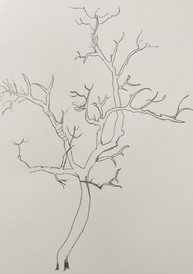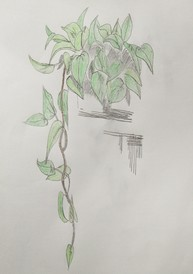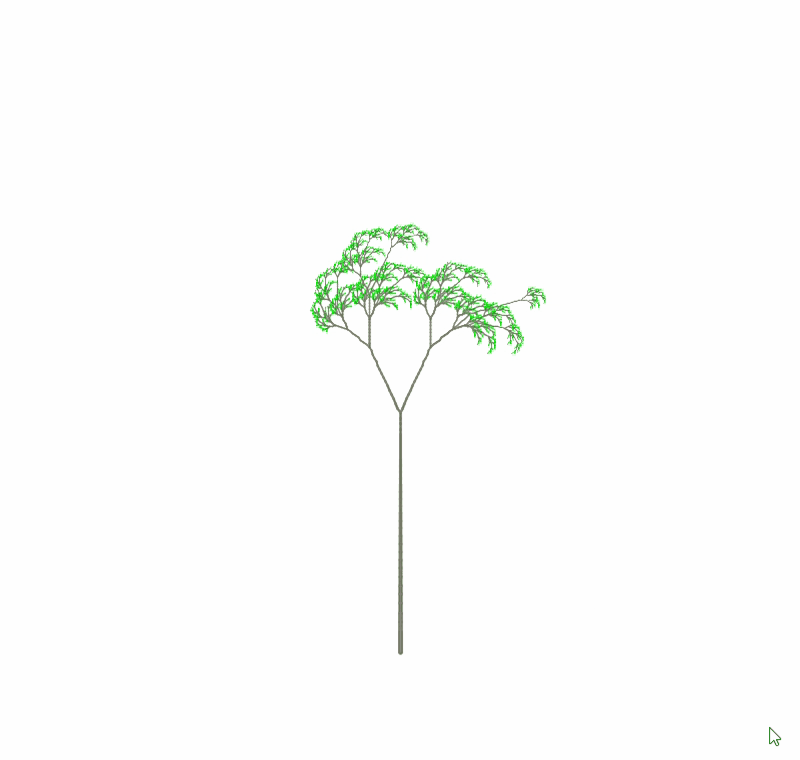A.技法：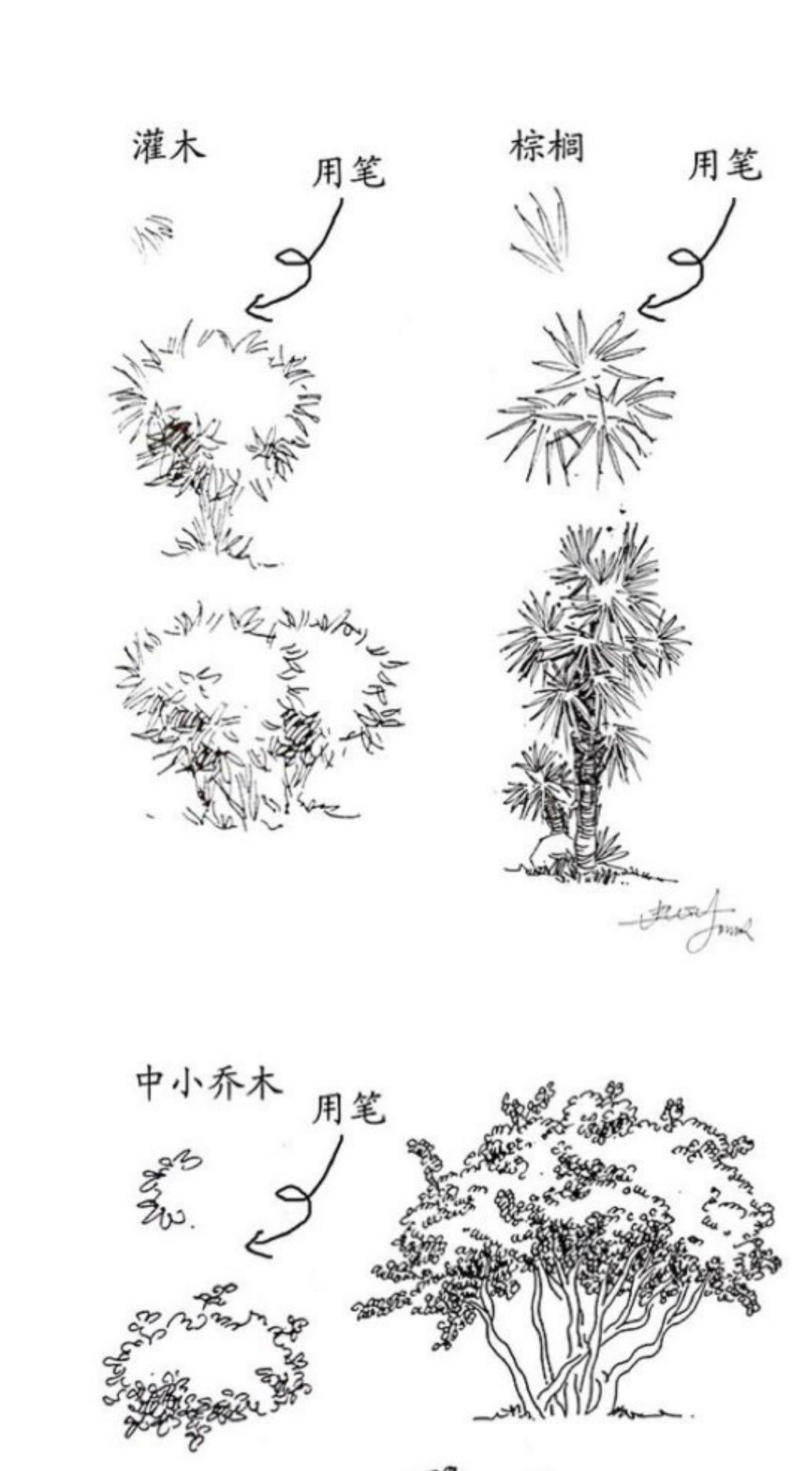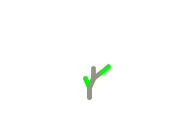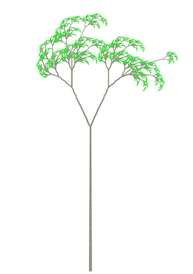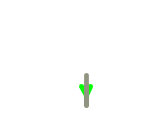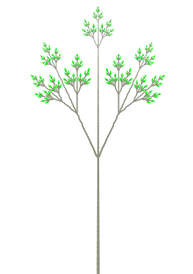B.理念

C.创作体验

D.呈现效果

E.局限性及应用

``````//生成构建树的字符串
class Grammar
{
string rules[];
int ruleNum=0;
int times;
chArr result;
char axioms;
Grammar(int t, string R[], char a)
{
this.ruleNum=R.length;
this.times=t;
result=new chArr();
rules=new string[R.length];
for (int k=0; k<R.length; k++)
{
//rules[k]=new string();
rules[k]=R[k];
}
axioms=a;
this.Iteration();
}

void Iteration()
{

for (int i=0; i<this.times; i++)
{
chArr tmpstr=new chArr();
for (int j=0; j<result.size; j++)
{
}
result .set(tmpstr);
}
}
chArr getResult()
{

return result;
}
chArr search(char target)
{
//string generations;
chArr tmp=new chArr();
//generations=new string(target);

for (int i=0; i<rules.length; i++)
{
if (rules[i].first==target)
{
int index;
index=(int)(random(1)*rules[i].size);
return rules[i].str.get(index);
}
}
return tmp;
}
}
``````
``````//构建树的节点
void getVertices()
{

chArr result=this.grammar.getResult();
int i=0;
int count =0;
float ang=90;
IntList outbrake=new IntList();
FloatList angleSign=new FloatList();
FloatList lengthSign=new FloatList();
State curS;
ArrayList<State> node1=new ArrayList<State>();
curS=firstState;
for (int loop=0; loop<result.size; loop++)
{
char ch=result.get(loop);
switch(ch) {
//move forward
case 'F':
{
PVector p=new PVector();
State cur=new State();

if (i==0)
{
p.set(400, 150);
cur.setStartPos(p);
cur.setAngle(90);
cur.Length=treeLen;
} else
{
cur.Length=len*lengthFactor;
len=cur.Length;
}
cur.setAngle(ang);
curS=cur;
stack.push(cur);
break;
}
case 'f':
{
PVector p1=new PVector();
State cur=new State();

if (i==0)
{
p1.set(400, 150);
cur.setStartPos(p1);
cur.setAngle(90);
cur.Length=treeLen;
} else
{
cur.Length=len*lengthFactor;
len=cur.Length;
}
cur.setAngle(ang);
curS=cur;
stack.push(cur);
break;
}

case '+':
ang=ang-(this.increment.y);
break;
case '-':
ang=ang+(this.increment.y);
break;
case '[':
{
angleSign.append(curS.angle);
lengthSign.append(curS.Length);
outbrake.append(0);
count++;
break;
}
case ']':
{
len=lengthSign.get(count-1);
ang=angleSign.get(count-1);
angleSign.remove(angleSign.size()-1);
lengthSign.remove(lengthSign.size()-1);
outbrake.append(1);
curS.level=1;
curS=node1.get(count-1);
node1.remove(count-1);
count--;

break;
}
default:
break;
}
i++;
}
}
``````

1.了解基于L-系统算法
: 基于L-系统和 Perlin 噪声函数的风吹树动模拟-文档
:基于L-系统和 Perlin 噪声函数的风吹树动模拟–原论文

2.了解perlin噪声
: Perlin在线讲座

3.代码画画
:用代码画画——搞艺术的学编程有啥用？
:开始第一幅“码绘”——以编程作画的基本方法
3.processing学习
: processing学习文档
: processing三维用法

2016-05-31 12:29:40 whuhan2013 阅读数 2178
• ###### 手绘漫画人体结构与比例入门基础教程

课程详细介绍了插画常用的软件SAI、PS，数位板的功能和使用技巧，以及插画漫画的基础技巧，包括五官、人体比例的分配，头发、眼睛、衣服的细节刻画，造型原创等，详实的展示了插画漫画的创作思路和过程。

3596 人正在学习 去看看 竹芝

### 效果图### 代码实现``````//获取离指定点最近的一个未绘制过的点
private Point getNearestPoint(Point p) {
if (p == null) return null;
//
int beginX = (p.x - add) >= 0 ? (p.x - add) : 0;
int endX = (p.x + add) < mSrcBmWidth ? (p.x + add) : mSrcBmWidth - 1;
int beginY = (p.y - add) >= 0 ? (p.y - add) : 0;
int endY = (p.y + add) < mSrcBmHeight ? (p.y + add) : mSrcBmHeight - 1;
//搜索正方形的上下边
for (int x = beginX; x <= endX; x++) {
if (mArray[x][beginY]) {
//标记当前点已经访问过
mArray[x][beginY] = false;
return new Point(x, beginY);
}
if (mArray[x][endY]) {
//标记当前点已经访问过
mArray[x][endY] = false;
return new Point(x, endY);
}
}
//搜索正方形的左右边
for (int y = beginY + 1; y <= endY - 1; y++) {
if (mArray[beginX][y]) {
//标记当前点已经访问过
mArray[beginX][beginY] = false;
return new Point(beginX, beginY);
}
if (mArray[endX][y]) {
//标记当前点已经访问过
mArray[endX][y] = false;
return new Point(endX, y);
}
}
}

return null;
}``````

``````   private Point mLastPoint = new Point(0, 0);
//获取下一个需要绘制的点
private Point getNextPoint() {
mLastPoint = getNearestPoint(mLastPoint);
return mLastPoint;
}``````

``````/**
* //绘制
* return :false 表示绘制完成，true表示还需要继续绘制
*/
private boolean draw() {

mPaint.setStyle(Paint.Style.STROKE);
mPaint.setColor(Color.BLACK);
//获取count个点后，一次性绘制到bitmap在把bitmap绘制到SurfaceView
int count = 100;
Point p = null;
while (count-- > 0) {
p = getNextPoint();
if (p == null) {//如果p为空，说明所有的点已经绘制完成
return false;
}
mTmpCanvas.drawPoint(p.x, p.y + offsetY, mPaint);
}
//将bitmap绘制到SurfaceView中
Canvas canvas = mSurfaceHolder.lockCanvas();
canvas.drawBitmap(mTmpBm, 0, 0, mPaint);
if (p != null)
canvas.drawBitmap(mPaintBm, p.x, p.y - mPaintBm.getHeight() + offsetY, mPaint);
mSurfaceHolder.unlockCanvasAndPost(canvas);
return true;
}``````

``````package com.hc.myoutline;

import android.content.Context;
import android.graphics.Bitmap;
import android.graphics.Canvas;
import android.graphics.Color;
import android.graphics.Paint;
import android.graphics.Point;
import android.util.AttributeSet;
import android.view.SurfaceHolder;
import android.view.SurfaceView;

/**
* Package com.hc.myoutline
* Created by HuaChao on 2016/5/27.
*/
public class DrawOutlineView extends SurfaceView implements SurfaceHolder.Callback {

private SurfaceHolder mSurfaceHolder;
private Bitmap mTmpBm;
private Canvas mTmpCanvas;
private int mWidth;
private int mHeight;
private Paint mPaint;
private int mSrcBmWidth;
private int mSrcBmHeight;
private boolean[][] mArray;
private int offsetY = 100;

private Bitmap mPaintBm;
private Point mLastPoint = new Point(0, 0);

public DrawOutlineView(Context context) {
super(context);
init();
}

public DrawOutlineView(Context context, AttributeSet attrs) {
super(context, attrs);

init();
}

private void init() {
mSurfaceHolder = getHolder();
mPaint = new Paint();
mPaint.setColor(Color.BLACK);
}

//设置画笔图片
public void setPaintBm(Bitmap paintBm) {
mPaintBm = paintBm;
}

//获取离指定点最近的一个未绘制过的点
private Point getNearestPoint(Point p) {
if (p == null) return null;
//
int beginX = (p.x - add) >= 0 ? (p.x - add) : 0;
int endX = (p.x + add) < mSrcBmWidth ? (p.x + add) : mSrcBmWidth - 1;
int beginY = (p.y - add) >= 0 ? (p.y - add) : 0;
int endY = (p.y + add) < mSrcBmHeight ? (p.y + add) : mSrcBmHeight - 1;
//搜索正方形的上下边
for (int x = beginX; x <= endX; x++) {
if (mArray[x][beginY]) {
mArray[x][beginY] = false;
return new Point(x, beginY);
}
if (mArray[x][endY]) {
mArray[x][endY] = false;
return new Point(x, endY);
}
}
//搜索正方形的左右边
for (int y = beginY + 1; y <= endY - 1; y++) {
if (mArray[beginX][y]) {
mArray[beginX][beginY] = false;
return new Point(beginX, beginY);
}
if (mArray[endX][y]) {

mArray[endX][y] = false;
return new Point(endX, y);
}
}
}

return null;
}

//获取下一个需要绘制的点
private Point getNextPoint() {
mLastPoint = getNearestPoint(mLastPoint);
return mLastPoint;
}

/**
* //绘制
* return :false 表示绘制完成，true表示还需要继续绘制
*/
private boolean draw() {

mPaint.setStyle(Paint.Style.STROKE);
mPaint.setColor(Color.BLACK);
//获取count个点后，一次性绘制到bitmap在把bitmap绘制到SurfaceView
int count = 100;
Point p = null;
while (count-- > 0) {
p = getNextPoint();
if (p == null) {//如果p为空，说明所有的点已经绘制完成
return false;
}
mTmpCanvas.drawPoint(p.x, p.y + offsetY, mPaint);
}
//将bitmap绘制到SurfaceView中
Canvas canvas = mSurfaceHolder.lockCanvas();
canvas.drawBitmap(mTmpBm, 0, 0, mPaint);
if (p != null)
canvas.drawBitmap(mPaintBm, p.x, p.y - mPaintBm.getHeight() + offsetY, mPaint);
mSurfaceHolder.unlockCanvasAndPost(canvas);
return true;
}
//重画
public void reDraw(boolean[][] array) {
if (isDrawing) return;
mTmpBm = Bitmap.createBitmap(mWidth, mHeight, Bitmap.Config.ARGB_8888);
mTmpCanvas = new Canvas(mTmpBm);
mPaint.setColor(Color.WHITE);
mPaint.setStyle(Paint.Style.FILL);
mTmpCanvas.drawRect(0, 0, mWidth, mHeight, mPaint);
mLastPoint = new Point(0, 0);
beginDraw(array);
}

private boolean isDrawing = false;

public void beginDraw(boolean[][] array) {
if (isDrawing) return;
this.mArray = array;
mSrcBmWidth = array.length;
mSrcBmHeight = array.length;

@Override
public void run() {
while (true) {
isDrawing = true;
boolean rs = draw();
if (!rs) break;
try {
sleep(20);
} catch (InterruptedException e) {
e.printStackTrace();
}
}
isDrawing = false;
}
}.start();
}

@Override
public void surfaceCreated(SurfaceHolder holder) {

}

@Override
public void surfaceChanged(SurfaceHolder holder, int format, int width, int height) {
this.mWidth = width;
this.mHeight = height;
mTmpBm = Bitmap.createBitmap(width, height, Bitmap.Config.ARGB_8888);
mTmpCanvas = new Canvas(mTmpBm);
mPaint.setColor(Color.WHITE);
mPaint.setStyle(Paint.Style.FILL);
mTmpCanvas.drawRect(0, 0, mWidth, mHeight, mPaint);
Canvas canvas = holder.lockCanvas();
canvas.drawBitmap(mTmpBm, 0, 0, mPaint);
holder.unlockCanvasAndPost(canvas);

mPaint.setStyle(Paint.Style.STROKE);
}

@Override
public void surfaceDestroyed(SurfaceHolder holder) {

}
}``````

``````package com.hc.myoutline;

import android.graphics.Bitmap;
import android.graphics.Color;
import android.os.Bundle;
import android.support.v7.app.AppCompatActivity;
import android.view.MotionEvent;

public class MainActivity extends AppCompatActivity {
private DrawOutlineView drawOutlineView;
private Bitmap sobelBm;

@Override
protected void onCreate(Bundle savedInstanceState) {
super.onCreate(savedInstanceState);

setContentView(R.layout.activity_main);
//将Bitmap压缩处理，防止OOM
Bitmap bm = CommenUtils.getRatioBitmap(this, R.drawable.test, 100, 100);
//返回的是处理过的Bitmap
sobelBm = SobelUtils.Sobel(bm);
drawOutlineView = (DrawOutlineView) findViewById(R.id.outline);

Bitmap paintBm = CommenUtils.getRatioBitmap(this, R.drawable.paint, 10, 20);
drawOutlineView.setPaintBm(paintBm);

}
//根据Bitmap信息，获取每个位置的像素点是否需要绘制
//使用boolean数组而不是int[][]主要是考虑到内存的消耗
private boolean[][] getArray(Bitmap bitmap) {
boolean[][] b = new boolean[bitmap.getWidth()][bitmap.getHeight()];

for (int i = 0; i < bitmap.getWidth(); i++) {
for (int j = 0; j < bitmap.getHeight(); j++) {
if (bitmap.getPixel(i, j) != Color.WHITE)
b[i][j] = true;
else
b[i][j] = false;
}
}
return b;
}

boolean first = true;

//点击时开始绘制
@Override
public boolean onTouchEvent(MotionEvent event) {
if (first) {
first = false;
drawOutlineView.beginDraw(getArray(sobelBm));
} else
drawOutlineView.reDraw(getArray(sobelBm));
return true;
}
}``````

### 参考链接

Android自动手绘，圆你儿时画家梦！ - huachao1001的专栏 - 博客频道 - CSDN.NET

2019-07-14 14:07:20 Hill_L 阅读数 66
• ###### 手绘漫画人体结构与比例入门基础教程

课程详细介绍了插画常用的软件SAI、PS，数位板的功能和使用技巧，以及插画漫画的基础技巧，包括五官、人体比例的分配，头发、眼睛、衣服的细节刻画，造型原创等，详实的展示了插画漫画的创作思路和过程。

3596 人正在学习 去看看 竹芝

1）导入图片；
2）将图片转换成矩阵或者数组格的格式。（原因在于图片以RGB格式保存在数组中）
3）通过数值运算改变RGB的值；（梯度
4）将调整后的数组值，重新生成一个图像文件；
5) 将图像文件保存即可。